Next: Particle in Finite Square Up: Wave Mechanics Previous: Stationary States

# Three-Dimensional Wave Mechanics

Up to now, we have only discussed wave mechanics for a particle moving in one dimension. However, the generalization to a particle moving in three dimensions is fairly straightforward. A massive particle moving in three dimensions has a complex wavefunction of the form [cf., Equation (1094)]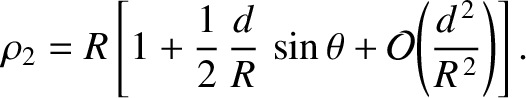(1154)

where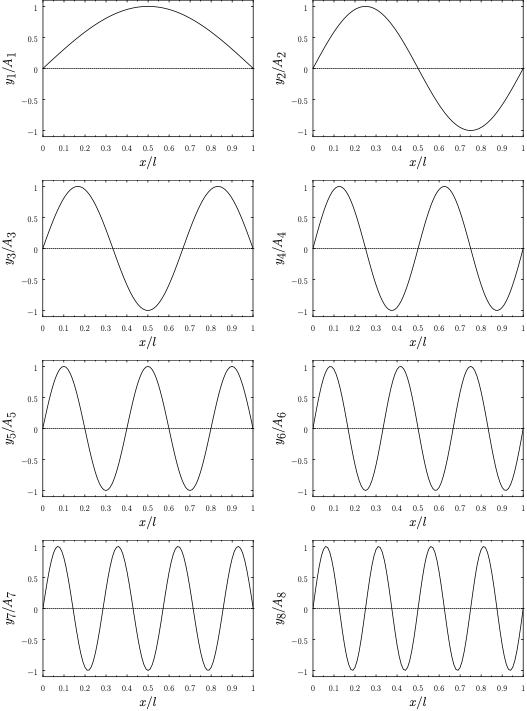is a complex constant, and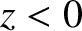. Here, the wavevector,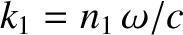, and the angular frequency,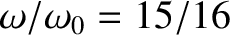, are related to the particle momentum,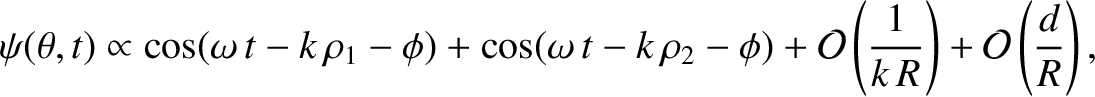, and energy,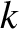, according to [cf., Equation (1082)]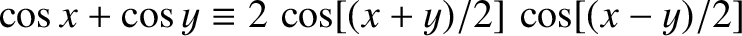(1155)

and [cf., Equation (1080)]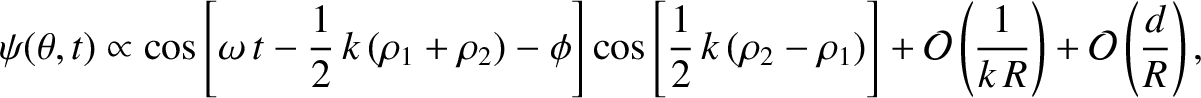(1156)

respectively. Generalizing the analysis of Section 12.5, the three-dimensional version of Schrödinger's equation is [cf., Equation (1102)]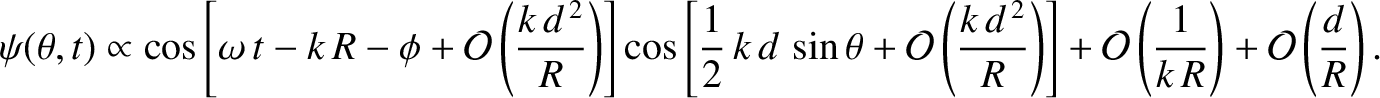(1157)

where the differential operator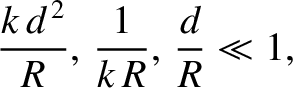(1158)

is known as the Laplacian. The interpretation of a three-dimensional wavefunction is that the probability of simultaneously finding the particle between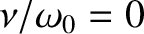and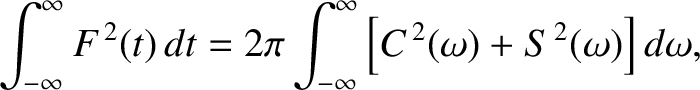, betweenand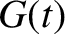, and betweenand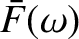, at time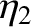is [cf., Equation (1105)]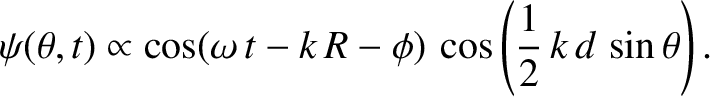(1159)

Moreover, the normalization condition for the wavefunction becomes [cf., Equation (1107)]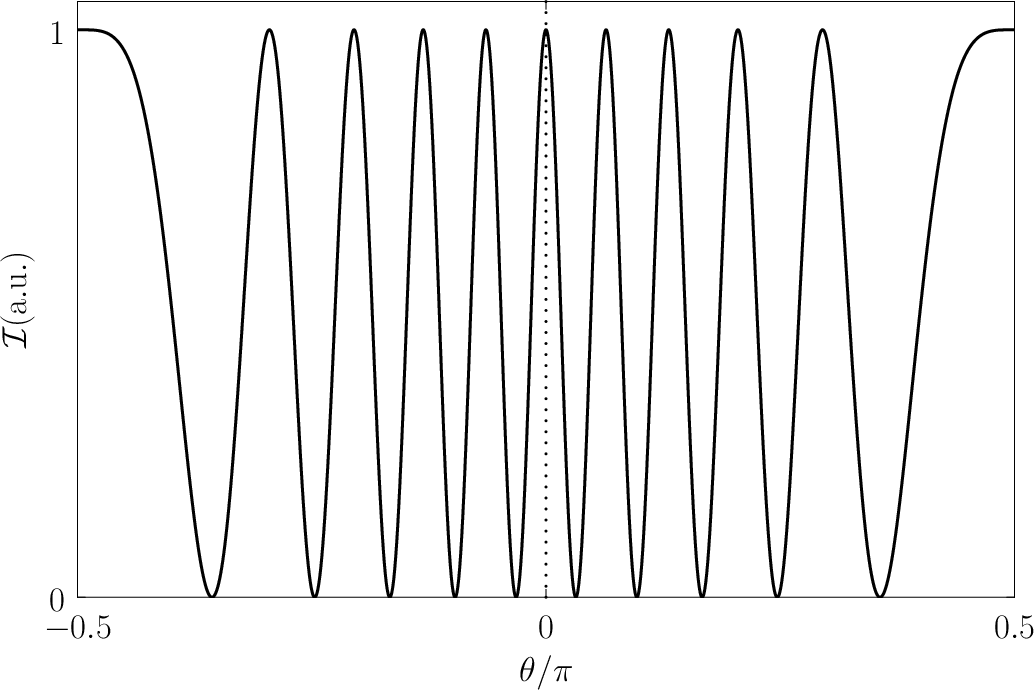(1160)

It can be demonstrated that Schrödinger's equation, (1157), preserves the normalization condition, (1160), of a localized wavefunction (Gasiorowicz 1996). Heisenberg's uncertainty principle generalizes to [cf., Equation (1135)]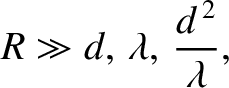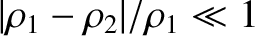(1161)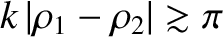(1162)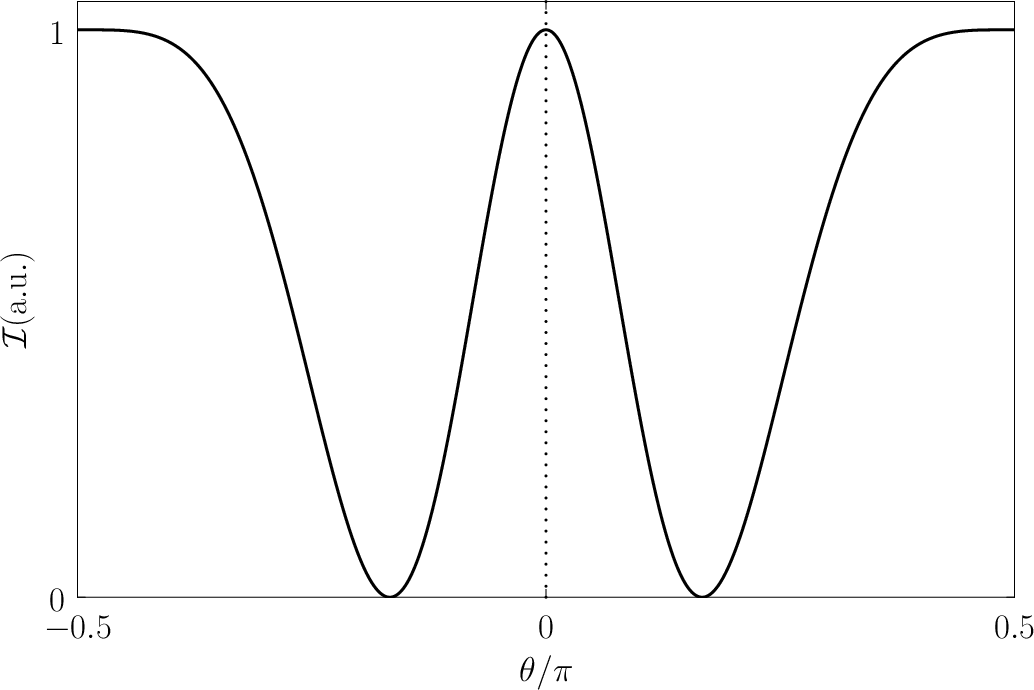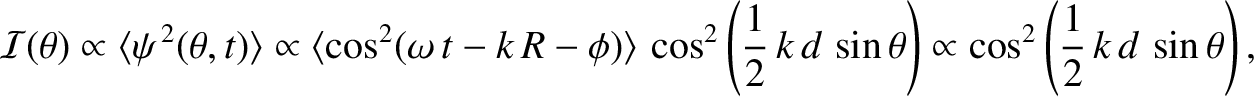(1163)

Finally, a stationary state of energyis written [cf., Equation (1139)]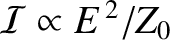(1164)

where the stationary wavefunction,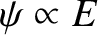, satisfies [cf., Equation (1141)]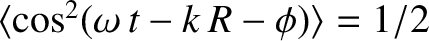(1165)

As an example of a three-dimensional problem in wave mechanics, consider a particle trapped in a square potential well of infinite depth, such that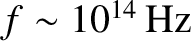(1166)

Within the well, the stationary wavefunction,, satisfies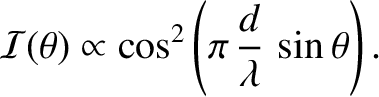(1167)

subject to the boundary conditions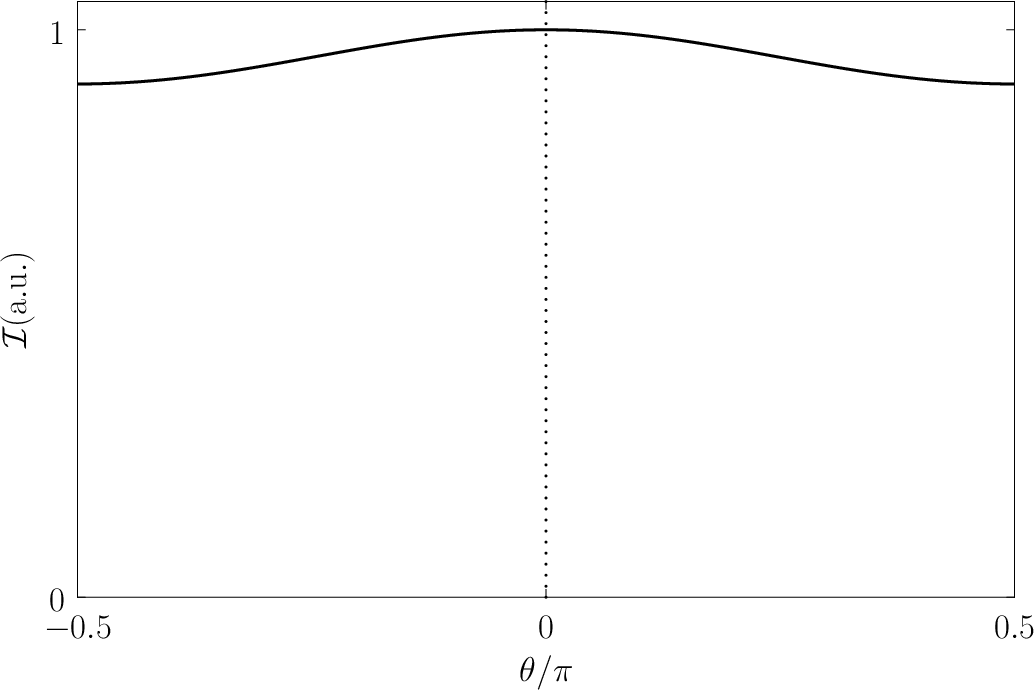(1168)

and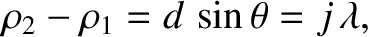(1169)

becauseoutside the well. Let us try a separable wavefunction of the form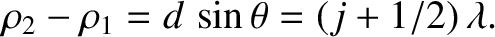(1170)

This expression automatically satisfies the boundary conditions (1168). The remaining boundary conditions, (1169), are satisfied provided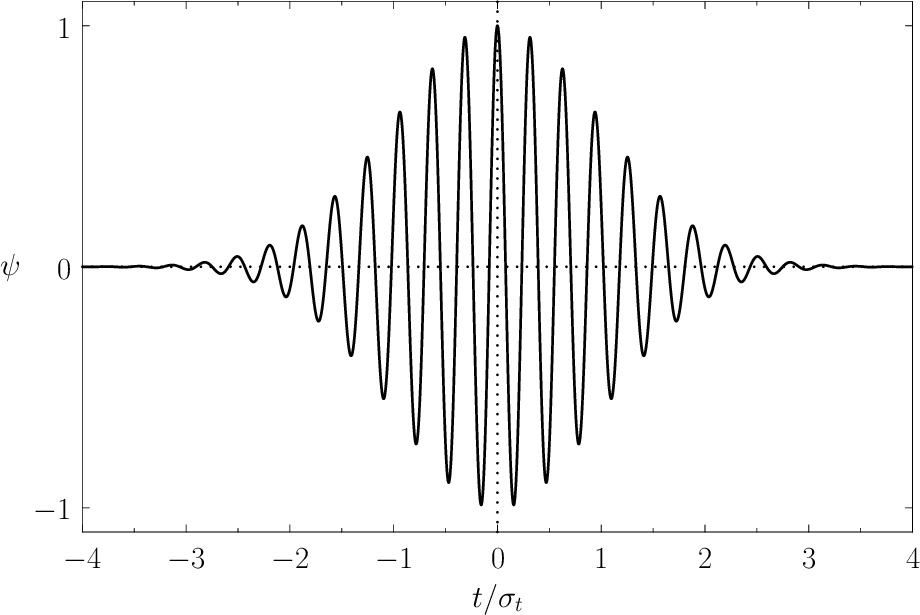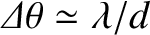(1171)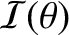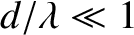(1172)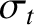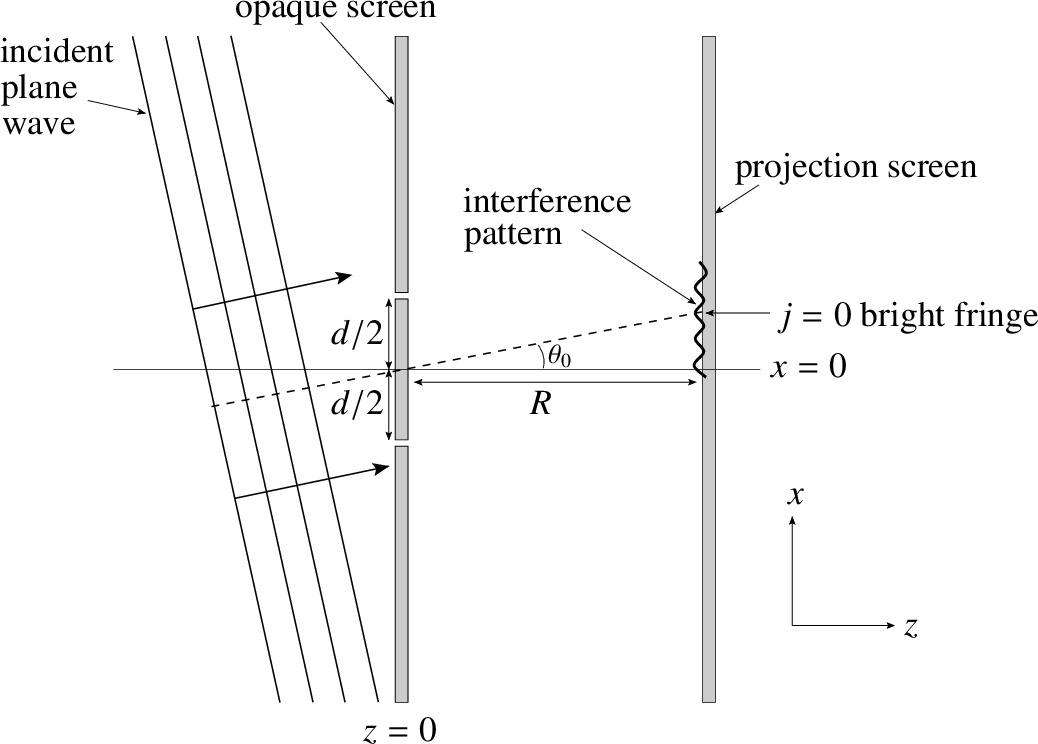(1173)

where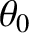,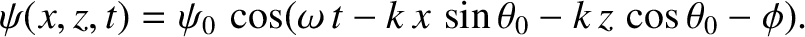, and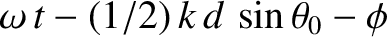are (independent) positive integers. Substitution of the wavefunction (1170) into Equation (1167) yields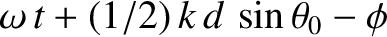(1174)

Thus, it follows from Equations (1171)-(1173) that the particle energy is quantized, and that the allowed energy levels are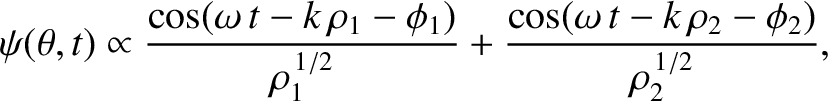(1175)

The properly normalized [see Equation (1160)] stationary wavefunctions corresponding to these energy levels are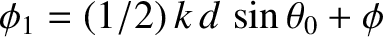(1176)

As is the case for a particle trapped in a one-dimensional potential well, the lowest energy level for a particle trapped in a three-dimensional well is not zero, but rather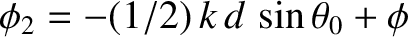(1177)

Here,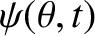(1178)

is the ground state (i.e., the lowest energy state) energy in the one-dimensional case. It follows from Equation (1175) that distinct permutations of,, andthat do not alter the value of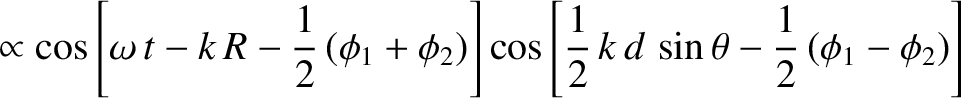also do not alter the energy. In other words, in three dimensions, it is possible for distinct wavefunctions to be associated with the same energy level. In this situation, the energy level is said to be degenerate. The ground state energy level,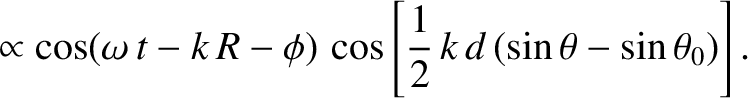, is non-degenerate, because the only combination of (,,) that gives this energy is (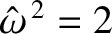,,). However, the next highest energy level,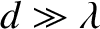, is degenerate, because it is obtained when (,,) take the values (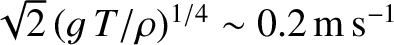,,), or (,,), or (,,). In fact, a non-degenerate energy level corresponds to a case where the three mode numbers (i.e.,,, and) all have the same value, whereas a three-fold degenerate energy level corresponds to a case where only two of the mode numbers have the same value, and, finally, a six-fold degenerate energy level corresponds to a case where the mode numbers are all different.Next: Particle in Finite Square Up: Wave Mechanics Previous: Stationary States
Richard Fitzpatrick 2013-04-08